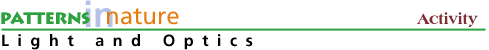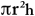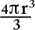Measurements and Estimates### Introduction

Ever wonder how many gumballs there really are in that gumball machine? Or how many seconds there are in a year? We often use simple estimation techniques to figure out how many of something there are or how long something lasts. In doing so, sometimes we also have to deal with rather large or small numbers. This activity will introduce the concepts of estimation, powers of ten, and the metric system, all of which are used everyday in science.

### Procedure

• Gather these items from your home or workplace:
• a centimeter ruler
• a stack of paper
• lens B or the Fresnel lens from your Optics Kit
• some salt
• a jelly bean
• a container to hold the jelly beans

• Estimate the thickness of a sheet of paper. Measure in centimeters the thickness of a stack of paper -- for example 200 sheets (400 numbered pages) in a book or a package of copy paper (usually 500 sheets per package). The thickness of each sheet should be about 100 microns where 1 micron = 1 micrometer = 10-6 meter = 1/10,000th centimeter (see Powers of Ten Reading for more info on conversions).

• Use your Optics Kit hand lens B or Fresnel lens to look at the thickness of the paper end on. Estimate the thickness of a human hair* and the width of a line of a letter in a book.

• Measure the volume of salt. Place salt crystals on a sheet of paper and compare them to the diameter of a hair. Salt crystals are cubic and have a volume of L3 where L is the edge length. Assume the average length L is 100 microns, then estimate the volume. How many salt crystals are in a container 10 centimeters high (h) and 2 centimeters in radius (r)?

volume of a cylinder =, where pi = 3.14

• Measure the volume of a jelly bean. With a centimeter ruler, estimate the volume of a jelly bean (assuming the shape of a cylinder) or a gumball
(spherical shape with volume =with r the radius)

by measuring r and h. Measure the volume of a container and estimate the number of jelly beans or gumballs it would hold. Then count them and see how close you come.

* The thickness of a human hair should be somewhat less than 100 microns, perhaps 70 microns